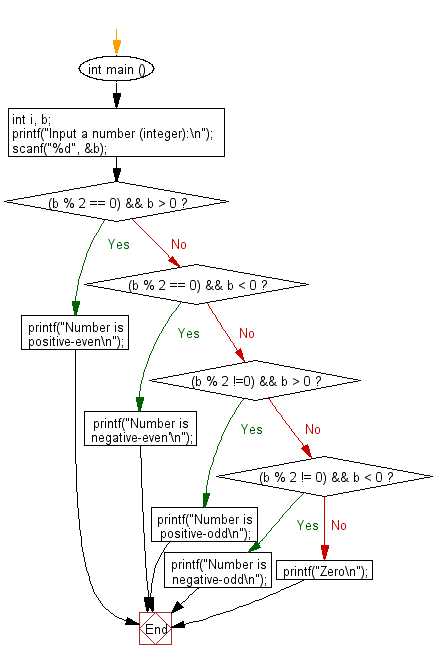﻿ C : From a number, get the odd, even, positive, and negative

# C Exercises: Find the odd, even, positive and negative number form a given number and print a message

## C Basic Declarations and Expressions: Exercise-110 with Solution

Write a C program to find the odd, even, positive and negative numbers from a given number (integer) and print a message 'Number is positive odd' or 'Number is negative odd' or 'Number is positive even' or 'Number is negative even'. If the number is 0 print "Zero".

Sample Solution:

C Code:

``````#include <stdio.h>
int main () {
int b;

// Prompt the user to input a number (integer)
printf("Input a number (integer):\n");

scanf("%d", &b);

// Check if the number is positive and even
if ((b % 2 == 0) && b > 0){
printf("Number is positive-even\n");
}
else{
// Check if the number is negative and even
if ((b % 2 == 0) && b < 0){
printf("Number is negative-even'\n");
}
else{
// Check if the number is positive and odd
if ((b % 2 !=0) && b > 0){
printf("Number is positive-odd\n");
}
else{
// Check if the number is negative and odd
if ((b % 2 != 0) && b < 0){
printf("Number is negative-odd\n");
}
else{
// If none of the above conditions are met, it must be zero
printf("Zero\n");
}
}
}
}
}
``````

Sample Output:

```Input a number (integer):
12
Number is positive-even
```

Flowchart:C programming Code Editor:

What is the difficulty level of this exercise?

Test your Programming skills with w3resource's quiz.

﻿